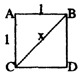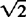﻿ Reductio ad Absurdum and the Square Root of Two - Cosmos - Carl Sagan ﻿

## Cosmos - Carl Sagan (1980)

### APPENDIX 1. Reductio ad Absurdum and the Square Root of Two

The original Pythagorean argument on the irrationality of the square root of 2 depended on a kind of argument called reductio ad absurdum, a reduction to absurdity: we assume the truth of a statement, follow its consequences and come upon a contradiction, thereby establishing its falsity. To take a modern example, consider the aphorism by the great twentieth-century physicist, Niels Bohr: “The opposite of every great idea is another great idea.” If the statement were true, its consequences might be at least a little perilous. For example, consider the opposite of the Golden Rule, or proscriptions against lying or “Thou shalt not kill.” So let us consider whether Bohr’s aphorism is itself a great idea. If so, then the converse statement, “The opposite of every great idea is not a great idea,” must also be true. Then we have reached a reductio ad absurdum. If the converse statement is false, the aphorism need not detain us long, since it stands self-confessed as not a great idea.

We present a modern version of the proof of the irrationality of the square root of 2 using a reductio ad absurdum, and simple algebra rather than the exclusively geometrical proof discovered by the Pythagoreans. The style of argument, the mode of thinking, is at least as interesting as the conclusion:Consider a square in which the sides are 1 unit long (1 centimeter, 1 inch, 1 light-year, it does not matter). The diagonal line BC divides the square into two triangles, each containing a right angle. In such right triangles, the Pythagorean theorem holds: 12 + 12 = X2. But 12 + 12 = 1 + 1 = 2, so X2 = 2 and we write x =the square root of two. We assumeis a rational number= p/q, where p and q are integers, whole numbers. They can be as big as we like and can stand for any integers we like. We can certainly require that they have no common factors. If we were to claim= 14/10, for example, we would of course cancel out the factor 2 and write p = 7 and q = 5, not p = 14, q = 10. Any common factor in numerator or denominator would be canceled out before we start. There are an infinite number of p’s and q’s we can choose. From= p/q, by squaring both sides of the equation, we find that 2 = p2/q2, or, by multiplying both sides of the equation by q2, we find

p2 = 2q2.     (Equation 1)

p2 is then some number multiplied by 2. Therefore p2 is an even number. But the square of any odd number is odd (12 = 1, 32 = 9, 52 = 25, 72 = 49, etc.). So p itself must be even, and we can write p = 2s, where s is some other integer. Substituting for p in Equation (1), we find

p2 = (2s)2 = 4s2 = 2q2

Dividing both sides of the last equality by 2, we find

q2 = 2s2

Therefore q2 is also an even number, and, by the same argument as we just used for p, it follows that q is even too. But if p and q are both even, both divisible by 2, then they have not been reduced to their lowest common factor, contradicting one of our assumptions. Reductio ad absurdum. But which assumption? The argument cannot be telling us that reduction to common factors is forbidden, that 14/10 is permitted and 7/5 is not. So the initial assumption must be wrong; p and q cannot be whole numbers; andis irrational. In fact,= 1.4142135 …

What a stunning and unexpected conclusion! How elegant the proof! But the Pythagoreans felt compelled to suppress this great discovery.

﻿
﻿